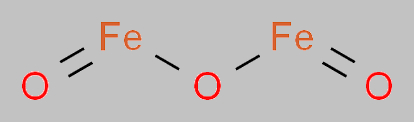# Iron (III) Oxide Formula

Iron (III) oxide formula is one of the common formulas that students come across while studying chemistry in several classes. Normally, the use of the formula and the reactions associated with it progress from basic to more complex as the students advance from a lower class to higher classes. Iron (III) oxide which is also known as ferric oxide is a chemical compound which is inorganic. It is one of the iron oxides which is important and it contains a mineral called hematite making it an essential source of iron. This compound is also sometimes referred to as rust or hydrated ferric oxide.

## Iron (III) Oxide Chemical Formula

The formula of iron (III) oxide is given as Fe2O3. The formula is derived by taking the valency of the compound. Generally, oxygen (O) has a valency of 2 whereas Iron (Fe) has a valency of 3. When we write the formula it is a common process where the compounds exchange valencies to attain a neutral and balanced state. Therefore, the valency of Oxygen goes to Iron and vice versa.

 Formula Fe2O3 Molar Mass 159.69 g/mol Density 5.242 g/cm3 Melting Point 1566 °C (Theoretical) Boiling Point Usually decomposes

### Iron (III) Oxide Structural Formula

The structural formula of iron(III) oxide can be represented as;Stay connected to BYJU’S to know more about other chemical compound formulas as well as different chemistry concepts.